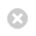Close

Short courses

Home# Introduction to Regression Analysis (Online, Self Paced)

• 6 hours
• No set start date, self-paced study.

## Overview

On this online, self-paced course you'll get an overview of regression types and learn about the application of multiple linear regression.

The main part of the course focuses on the theory behind regression analysis, in particular linear regression, and covers the formulation, interpretation and validation of linear regression models.

This course is delivered by UCL's Centre for Applied Statistics Courses (CASC), part of the UCL Great Ormond Street Institute of Child Health (ICH).

## Course content

Regression is a vital tool for any quantitative researcher. This course takes you from the basics of types of regression to the formulation of a multiple linear regression model. Interaction terms are introduced and explained.

Regression analysis is a very powerful technique that allows you to investigate the combined associations between one or more predictors and an outcome. You will learn about some examples where this is helpful:

• where within a trial you may wish to adjust for factors that differ between treatment groups to gauge the true effect of treatment
• in observational studies where you might want to take into account differences between the demographics or health behaviours of two or more subgroups
• when considering the combined effects of different factors, which may facilitate understanding of variation in outcome.

## Learning outcomes

You'll gain an overview of different regression types, and have the tools to perform and interpret the results from one specific type named 'linear regression'. The main course focuses on the theory behind regression analysis, and covers the formulation, interpretation and validation of linear regression models. In particular, you'll be able to:

• detail the basic principles behind regression analysis
• identify which type of regression is appropriate for a given research question and context
• perform a simple linear regression analysis, and be able to display graphically and interpret the output
• explain the merits of multiple regression and perform such an analysis
• include and interpret interaction terms in a model
• assess the assumptions of linear regression and interpret whether the fitted model is valid
• decide on a final model by considering potential variables for inclusion

## Cost and concessions

The standard price is £75.

A 50% discount is available for UCL staff, students, alumni. If you're eligible for a discount, email ich.statscou@ucl.ac.uk before booking to be sent the discount code.

## Certificates

You can download a Certificate of Participation when you have completed all the session quizzes.

## Find out about other statistics courses

CASC's stats courses are suitable for anyone requiring an understanding of research methodology and statistical analyses. The courses allow non-statisticians to interpret published research and/or undertake their own research studies.

Find out more about CASC's full range of statistics courses